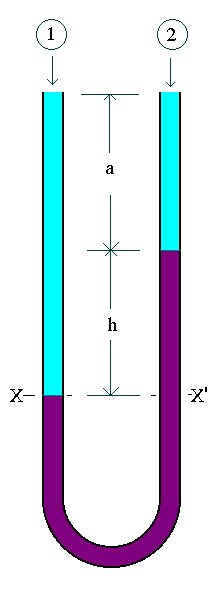### Simple U - tube Manometer

Home -> Lecture Notes -> Fluid Mechanics -> Unit-IEquating the pressure at the level XX'(pressure at the same level in a continuous body of fluid is equal),

For the left hand side:

Px = P1 + rg(a+h)

For the right hand side:

Px' = P2 + rga + rmgh

Since Px = Px'

P1 + rg(a+h) = P2 + rga + rmgh

P1 - P2 = rmgh - rgh

i.e. P1 - P2 = (rm - r)gh.
The maximum value of P1 - P2 is limited by the height of the manometer. To measure larger pressure differences we can choose a manometer with heigher density, and to measure smaller pressure differences with accuracy we can choose a manometer fluid which is having a density closer to the fluid density.